Test: Matrices & Determinants - 5

# Test: Matrices & Determinants - 5

Test Description

## 20 Questions MCQ Test IIT JAM Mathematics | Test: Matrices & Determinants - 5

Test: Matrices & Determinants - 5 for Mathematics 2023 is part of IIT JAM Mathematics preparation. The Test: Matrices & Determinants - 5 questions and answers have been prepared according to the Mathematics exam syllabus.The Test: Matrices & Determinants - 5 MCQs are made for Mathematics 2023 Exam. Find important definitions, questions, notes, meanings, examples, exercises, MCQs and online tests for Test: Matrices & Determinants - 5 below.
Solutions of Test: Matrices & Determinants - 5 questions in English are available as part of our IIT JAM Mathematics for Mathematics & Test: Matrices & Determinants - 5 solutions in Hindi for IIT JAM Mathematics course. Download more important topics, notes, lectures and mock test series for Mathematics Exam by signing up for free. Attempt Test: Matrices & Determinants - 5 | 20 questions in 20 minutes | Mock test for Mathematics preparation | Free important questions MCQ to study IIT JAM Mathematics for Mathematics Exam | Download free PDF with solutions
 1 Crore+ students have signed up on EduRev. Have you?
Test: Matrices & Determinants - 5 - Question 1

### If A =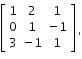then

Test: Matrices & Determinants - 5 - Question 2

### The value of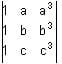is λ(a – b) (b – c) (c – a), where λ is equal to

Test: Matrices & Determinants - 5 - Question 3

### If A and B are two non-zero square matrices such that AB = 0, then

Test: Matrices & Determinants - 5 - Question 4

If the system of equations x - ky - z = 0, kx - y - z = 0, x + y - z = 0 has a non-zero solution, then the possible values of k are

Test: Matrices & Determinants - 5 - Question 5

The number of distinct real roots of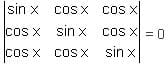in the interval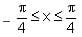is

Test: Matrices & Determinants - 5 - Question 6

If for a triangle ABC,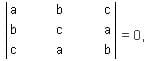then what is the value of sin3 A + sin3 B + sin3 C?

Test: Matrices & Determinants - 5 - Question 7

If the system of equations x - Ky - z = 0, Kx - y - z = 0 and x + y - z = 0 has a non-zero solution, then the possible values of K are

Test: Matrices & Determinants - 5 - Question 8

If a > 0 and discriminant of ax2 + 2bx + c is negative, then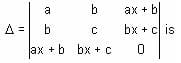Test: Matrices & Determinants - 5 - Question 9

If A is an invertible matrix and B is a matrix, then

Test: Matrices & Determinants - 5 - Question 10

If every minor of order r of a matrix A is zero, then rank of A is

Test: Matrices & Determinants - 5 - Question 11

The system of equations kx + y + z = 1, x + ky + z = k and x + y + kz = k2 have no solution if k equals

Test: Matrices & Determinants - 5 - Question 12

For what value of λ, the system of equations x - 2y + z = 0, 2x - y + 3z = 0, x + y - z = 0 has the trivial solution as the only solution.

Test: Matrices & Determinants - 5 - Question 13

If x ≠ y ≠ z and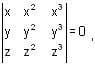then xyz is equal to

Test: Matrices & Determinants - 5 - Question 14

For a≠ 0 (I = 1, 2, 3, ……., n) the sum of co-factors of all elements of the determinant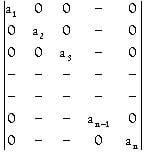for all n is

Test: Matrices & Determinants - 5 - Question 15

The system of equations x + 2y - z = 3, 2x - 2y + 3z = 2, 3x - y + 2z = 1 has

Test: Matrices & Determinants - 5 - Question 16

If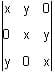= 0 and x ≠ 0, then

Test: Matrices & Determinants - 5 - Question 17

The determinant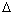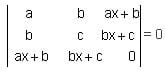Test: Matrices & Determinants - 5 - Question 18

The value of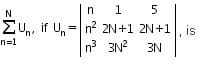Test: Matrices & Determinants - 5 - Question 19

The system of equations - 2x + y + z = a, x - 2y + z = b, x + y - 2z = c has no solution, if

Test: Matrices & Determinants - 5 - Question 20

If  d(r)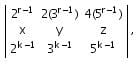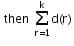is equal to

## IIT JAM Mathematics

106 videos|27 docs|30 tests
Information about Test: Matrices & Determinants - 5 Page
In this test you can find the Exam questions for Test: Matrices & Determinants - 5 solved & explained in the simplest way possible. Besides giving Questions and answers for Test: Matrices & Determinants - 5, EduRev gives you an ample number of Online tests for practice

## IIT JAM Mathematics

106 videos|27 docs|30 tests(Scan QR code)